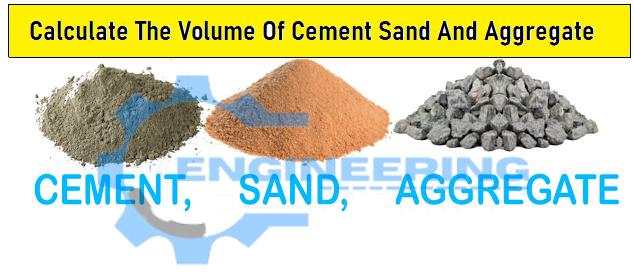Civil Engineering

# Calculate The Volume Of The Cement Sand And Aggregate

## Calculate The VolumeOf Cement SandAnd Aggregate

In this post i will discuss about the calculation of soncrete materials.Calculate The Volume Of Cement Sand And Aggregate.Volume Of Cement Sand and Aggregate For Slab |Volume Of Cement Sand and Aggregate For Beam| Volume Of Cement Sand And Aggregate For Column

when we find the volume of any objects its means the we use three dimesions Length, Height, Width or Breadth.The multiplication of these three quantites is equal to volume of that object.
We covered some Basic Surface Area and Volume Formulas.

### For Slab

lets suppose we have three dimesion
Length =5m
thickness = 0.125
Volume =length×breadth×Thickness = 5 ×3 ×O.125 = 3.75m³

### For Beam

lets suppose we have three dimesion
Length =5m
thickness = 0.3m
Volume =length×breadth×Thickness = 5 x 0.6 x 0.3 = 3.75 m³

### For Column

lets suppose we have three dimesion
Length =5m
thickness = 0.125m
Rectangular Column,
Volume = Height×Breadth×Width = 5 X 0.6 X 0.3 = 0.9 m³

### For Circular Column

height= 0.35m
Volume = πr²×h= 3.141256 x (0.15)2 X 5 = 0.35 m³
Now the Concrete Ingredients Calculation
For Cement, Sand and Coarse Aggregate.
This is a Volumetric Calculation.
supoose we need 2.000m³ of concrete for M20 Concrete Mix (Mix Ratio, M20 = 1 : 1.5 : 3)

some of concrete ratio= 1 + 1.5 + 3 = 5.5

NOW Cement Quantity = Cement / Sum of ratio ) x total volume of Concrete
Cement = 1/5.5 x total volume of Concrete = (1/5.5)x2 = 0.3636 m³

we know the Density of Cement = 1440 kg/ m³ = 0.3636 X 1440 = 523 kg = 10.5 says 11 bags

Sand Quantity = ( Sand / sum of ratio)xtotal volume of Concrete
Sand =(1.5/5.5) x 2 = 0.5454 m³
Coarse Aggregate Quantity = (Coarse Aggregate / Sum of ratio) x total volume of Concrete

Coarse Aggregate = (3/5.5) * 2 = 1.09 m³

### Water Cement Ratio

AS per standard (IS 10262 (2009), Water-Cement Ratio for the Concrete as 0.45
Requried of Amount of Water =Water Cement Ratio X Volume of Cement
Amount Of Water = 0.45×0.3636 m³ = 0.16362 m³
we Know
Unit Weight of Water = 1000 litres/m³
Required of Amount of Water = 0.16362 X 1000 = 163.62 litres
Now complete the Quantites of all materials
we need 11 bags of cement, 0.5454 m³ of sand, 1.09 m³ of Coarse aggregates and 163.62 litres of water.